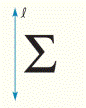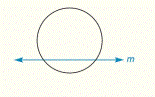Chapter 2.CR, Problem 46CR### Elementary Geometry for College St...

6th Edition
Daniel C. Alexander + 1 other
ISBN: 9781285195698

#### Solutions

Chapter
Section### Elementary Geometry for College St...

6th Edition
Daniel C. Alexander + 1 other
ISBN: 9781285195698
Textbook Problem
1 views

# Complete the drawing so that the figure is reflected across a) line l b) line mTo determine

a)

To draw:

The line of reflection across the line 𝓁.

Explanation

Given:

The given figure is,

Figure (1)

Property used:

With the reflection, every point of the original figure is reflected across a line in such a way as to make the given line a line of symmetry. Each pair of corresponding points will lie on opposite sides of the line of reflection and at equal distances.

Approach:

The drawing so that the Figure (1) is reflected across the line

To determine

(b)

To draw:

The line of reflection across the line m.

### Still sussing out bartleby?

Check out a sample textbook solution.

See a sample solution

#### The Solution to Your Study Problems

Bartleby provides explanations to thousands of textbook problems written by our experts, many with advanced degrees!

Get Started

#### Find more solutions based on key concepts